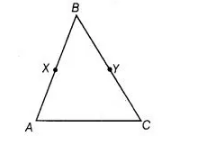`
Question:

In the adjoining figure, if AB = BC and BX = BY, then show that AX = CYSolution:

We have, AB = BC …(i)

and BX = BY ,.,.(ii)

According to Euclid’s axiom, if equals are subtracted from equals, the remainders are equal. So, on subtracting Eq. (ii) from Eq. (i), we get

AB- BX = BC – BY

=> AX = CY [from figure]